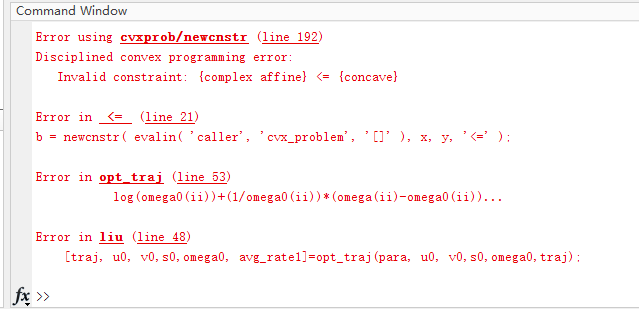# What should I do when the status is ‘Infeasible’ in cvx?

The code as following;
cvx_begin
variable traj(para.T+1, 2)
variable u(1, para.T)
variable v(1, para.T)
variable s(1, para.T)
variable omega(1, para.T)
expression avg_rate(1, para.T)

for ii=1:para.T
avg_rate(ii) =log(1+gamma0* C* 100*(1/norm([0,0,40]…
-para.place_user))*(para.kappa/(para.kappa+1)*omega(ii)…
+1/(para.kappa+1) * omega(ii))+D * (para.kappa/(para.kappa+1)*v(ii)…
+1/(para.kappa+1)*v(ii)))/log(2);
end

maximize 1/(para.T)*sum(avg_rate);

subject to
40960000 <= u;
1600<= v;
for ii=1:para.T
s(ii)<=s0(ii)+(3* traj0(ii,1)^2)* (traj(ii,1)-traj0(ii,1));
end

for ii=1:para.T
pow_pos(norm(traj(ii, :)-para.place_user(1:2)),2)+…
6400-v0(ii)^(-4/para.kappa)+…
4/para.kappa*(v0(ii)^(-4/(para.kappa)-1))*(v(ii)-v0(ii))<=0;
end

for ii=1:para.T
pow_pos(norm(traj(ii,:)-para.place_IOS(1:2)),2)+1600<=u(ii)^(1/2);
end

for ii=1:para.T
log(omega0(ii))+1/omega0(ii)* (omega(ii)-omega0(ii))…
+log(u0(ii))-1/u0(ii)* (u(ii)-u0(ii))…
<=log(s(ii));
end

for ii=1:para.T
pow_pos(norm(traj(ii+1,:)-traj(ii, :)),2)<=para.Vmax^2;
end

traj(para.T+1, :)==para.UAV_end(1:2);

cvx_end

Except for section 1, this also applies to CVX. https://yalmip.github.io/debugginginfeasible/

Dear Mark，
Thank you very much for the website you recommended to me. But I still have some questions.
I removed the objective functions that only run relevant restrictions. I found that if I delete the 7, the result will be solved. But the program will report an error.After reporting an error, I query the Omega value, It’s a negative number. That’s not possible, the OMEGA I defined can only be a positive number. Now that I know the errors of these two restrictions, how do I fix them?

Are there one or more complex numbers in the input data? Otherwise, how is anything `complex affine`?

I don’t know what or under hat circumstances your querying of `omega` consists of. Even if CVX reports the problem as solved or inaccurate solved (otherwise its value is meaningless), why should it be nonnegative? Where is it constrained to be nonnegative? Even if solved, and if constrained to be nonnegative, it could still be negative by an amount within solver feasibility tolerance of zero.

I want to make my objective function concave, so I use Omega to relax the division of the original two variables. A variable s is the absolute value, and a variable u is the distance. So I used to think that omega was non-negative.

Here is the code to report an error. I’ve made this mistake before, where the omega is zero.
for ii=1:para.T
log(omega0(ii))+1/omega0(ii)* (omega(ii)-omega0(ii))…
+log(u0(ii))-1/u0(ii)* (u(ii)-u0(ii))…
<=log(s(ii));
end
I just started with CVX and convex optimization. So I’m a little bit dull. Although my problem has not been solved, I will continue to work hard. Thank you for your always patient answer. To tell you the truth, each of your answers can make me happy all day.

Let’s say `s/u = 1`. How would the inequality constraint `omega <= s/u` prevent `omega` from being negative?